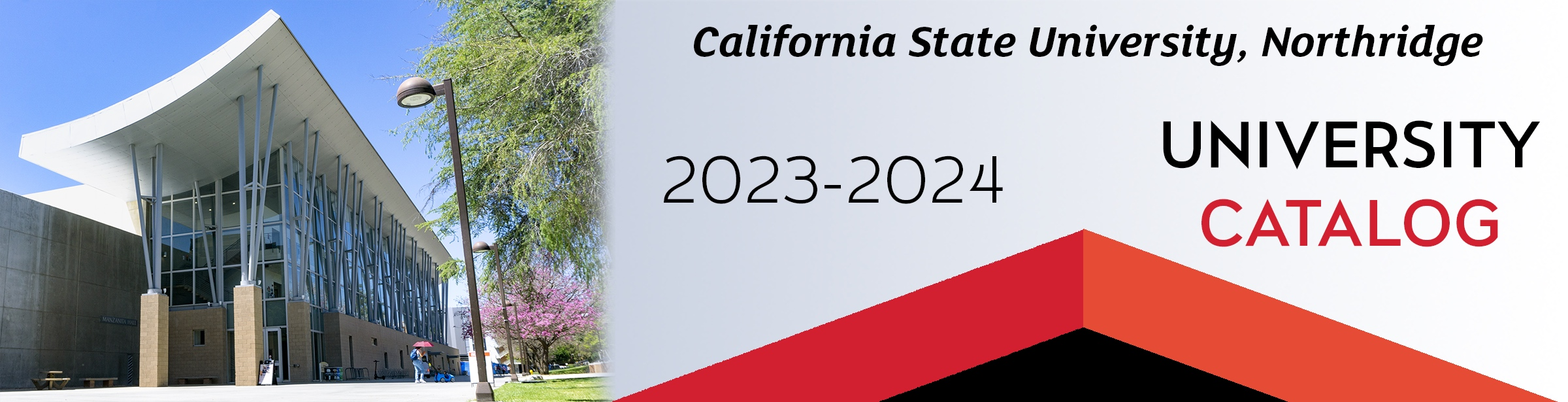## Course: ME 309. Numerical Analysis of Engineering Systems (2)

Prerequisites: MATH 150B; ME 209 or COMP 110/L or ECE 206/L. Review of technical computing and control flow programming fundamentals. Solution of a variety of non-trivial engineering problems through the use of modern analysis tools. Discussion of numerical methods covering nonlinear algebraic equations, linear algebraic systems of equations, eigenvalue problems, regression and curve fitting, numerical differentiation and integration and ordinary differential equations. Two 3-hour labs per week.

### ME 309

Class NumberLocationDayTime